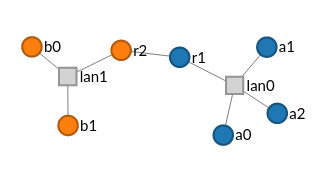# Network Emulation

This example shows how to set up network emulation in your experiment. In the topology depicted below, there are 2 enclaves distinguished by color. Each enclave is locally interconnected through an emulated multipoint link, and the connection between enclaves is an emulated point to point link.Emulation characteristics enter the model via capacity and latency constraints that are placed on links when they are created. These constraints are imported from the `mergexp.net` library.

from mergexp.net import capacity, latency
net.connect(nodes, capacity == mbps(100), latency == ms(10))

Link constraints are applied in the same way to point to point and multipoint links.

### Latency#

The behavior of latency round trip. So if you specify a latency of 10 milliseconds on a point to point link the delay will be 5 milliseconds in each direction.

### Capacity#

Capacity constraints are full-duplex. So if you specify a capacity of 100 mbps, the link will have that capacity in each direction.

Link parameters can be dynamically changed during the experiment lifetime through the use of the `moacmd` user tool on an XDC attached to the experiment.

##### note

In order to be able to change a link's parameters, the link must have one or more tags applied to in the topology model so that the user can specify which link is to be changed via `moacmd`. This is demonstrated in the full example below.

#### Usage#

moacmd set <tag> <param> <value>
• tag is the set of links with the specified tag applied in the XIR
• param is the link parameter to change (currently allowed: `bandwidth`, `delay`, `loss`)
• value is the value of param

This will set the parameter defined by param to the value of value on all links tagged with tag in the XIR.

##### Delay#

In the example below the latency between the two routers could be changed to 47 milliseconds follows.

moacmd set wan delay 47ms
##### Bandwidth#

Setting bandwidth to 47 megabits per second

moacmd set wan bandwidth 47mbps
##### Loss#

Setting loss rate to 4.7 percent

moacmd set wan loss 0.047

The following unit suffixes may be used

unitsuffix
delay`ns`, `us`, `ms`, `s`
bandwidth`bps`, `kbps`, `mbps`, `gbps`

### Complete Example#

import mergexp as mx
from mergexp.net import capacity, latency, routing, static, addressing, ipv4
from mergexp.unit import mbps, ms
net = mx.Topology('dumbbell', addressing == ipv4, routing == static)
a = [net.device(name) for name in ['a0', 'a1', 'a2']]
b = [net.device(name) for name in ['b0', 'b1']]
r = [net.device(name) for name in ['r1', 'r2']]
enclave1 = net.connect(a + [r], capacity == mbps(100), latency == ms(10))
enclave2 = net.connect(b + [r], capacity == mbps(200), latency == ms(20))
wan = net.connect(r, capacity == mbps(10), latency == ms(70))
wan.props['tags'] = ['wan', 'r0-r1']
for e in enclave1.endpoints:
e.endpoint.device.props['group'] = 1
for e in enclave2.endpoints:
e.endpoint.device.props['group'] = 2
mx.experiment(net)